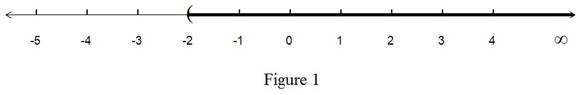# The inequality in terms of intervals and illustrate the solution set on real time line.### Single Variable Calculus: Concepts...

4th Edition
James Stewart
Publisher: Cengage Learning
ISBN: 9781337687805### Single Variable Calculus: Concepts...

4th Edition
James Stewart
Publisher: Cengage Learning
ISBN: 9781337687805

#### Solutions

Chapter A, Problem 11E
To determine

## To solve: The inequality in terms of intervals and illustrate the solution set on real time line.

Expert Solution

The value for the given expression is   x(2,).

### Explanation of Solution

Given:

The expression is 2x+7>3.

Calculation:

The expression can be re written as,

2x+7>32x>372x>4x>42

x>2

Therefore, x(2,).

Thus, the equivalent form of the inequality in the interval notation is x(2,).

The interval (2,) is shown below in Figure 1From Figure 1, it is observed that the value of x belongs to the darkened region in the number line.

### Have a homework question?

Subscribe to bartleby learn! Ask subject matter experts 30 homework questions each month. Plus, you’ll have access to millions of step-by-step textbook answers!